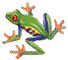## Chapitre 2 Les nombres de 0 à 69

Directions: Fill in each blank with the correct answer. After you fill in a blank, click anywhere on the page to check your answer. All the incorrect letters will be erased leaving only the part of your answer which is correct. Click on the ? button to see the correct answer. (If you have typed in the correct answer, nothing will change.) In order to type in an answer, the cursor must be visible inside the blank. Use the TAB key or the mouse.

Écrivez le résultat en toutes lettres. modèle: 1+1= deux

1. 2+3=

2. 3x5=

3. 49+1=

4. 1+2=

5. 10+3=

6. 29+1=

7. 30+3=

8. 1+1=

9. 6x2=

10. 17+3=

11. 20+1=

12. 20+2=

13. 2+2=

14. 10+4=

15. 38+2=

16. 0+6=

17. 15+1=

18. 6x10=

19. 70-1=

20. 40+1=